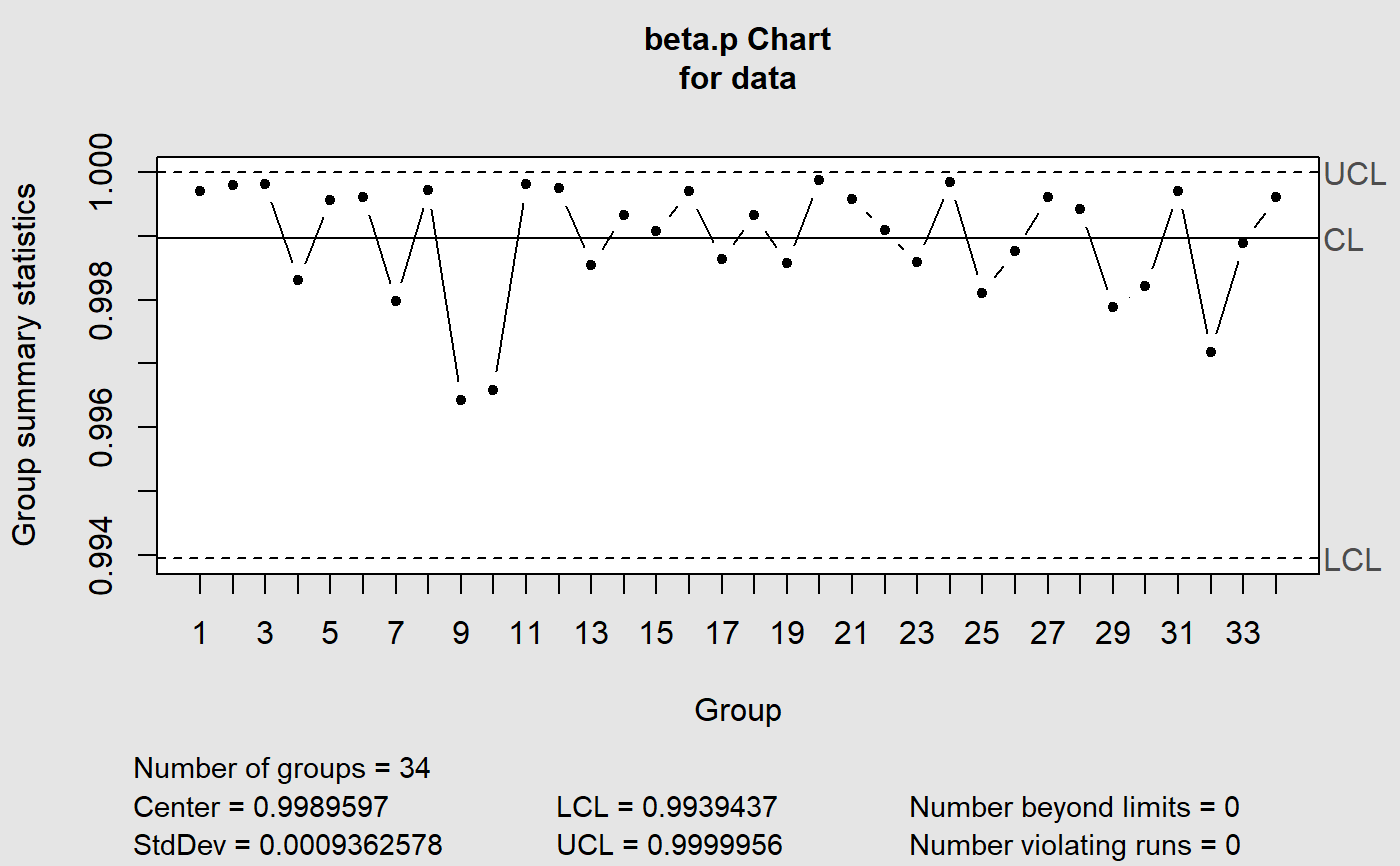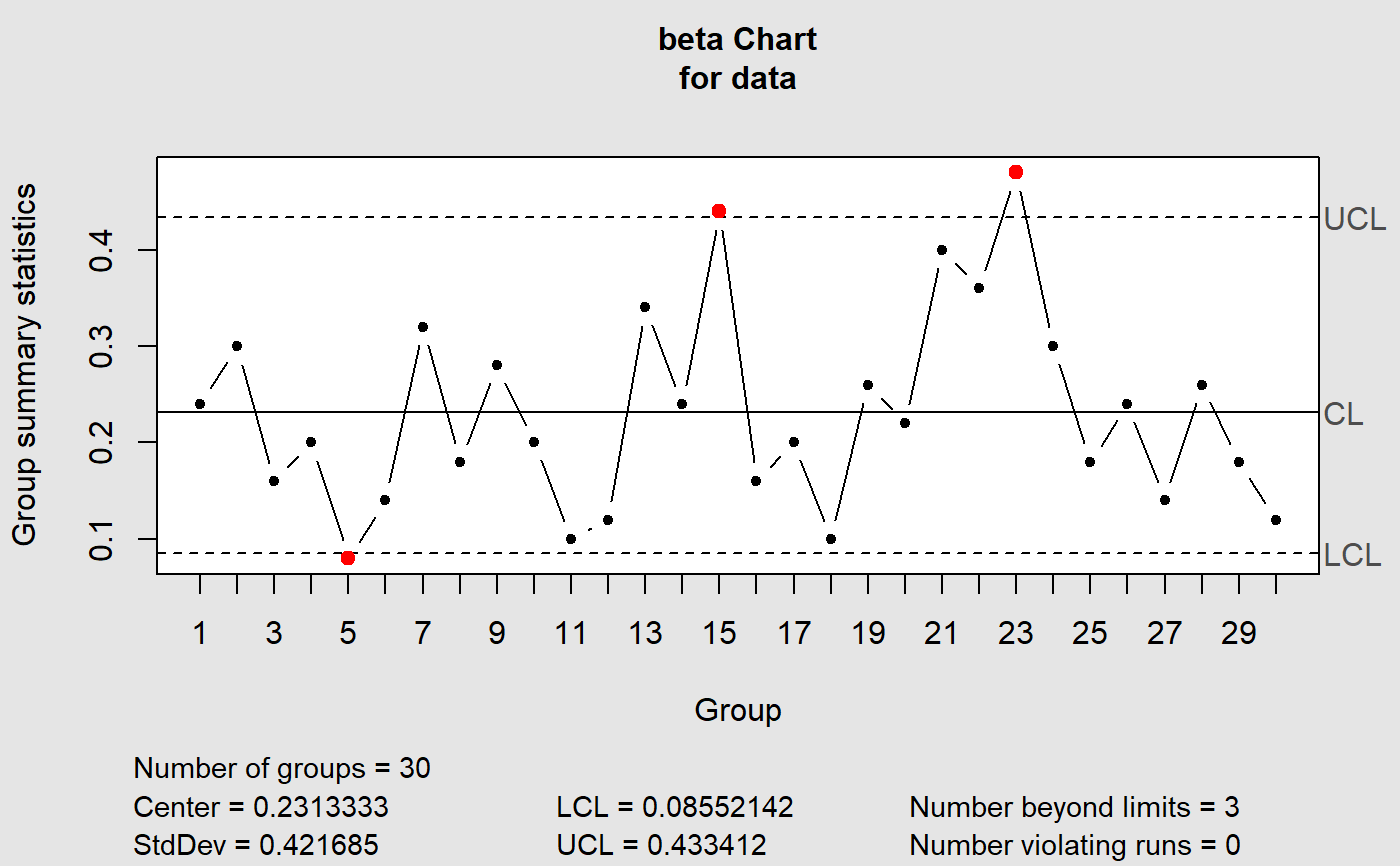Create an object of class 'qcc' to perform statistical quality control. This object may then be used to plot Beta Control Charts.

bcc(data, type = c("1", "2"), sizes, center, std.dev, limits, data.name,
labels, newdata, newsizes, newdata.name, newlabels, nsigmas = 3,
confidence.level = 0.9, rules = shewhart.rules, plot = TRUE, ...)

## Arguments

data a data frame, a matrix or a vector containing observed data for the variable to chart. Each row of a data frame or a matrix, and each value of a vector, refers to a sample or ''rationale group''. a character string specifying the group statistics to compute. There are two possible types: 1 for discrete data and 2 for continuous data. a value or a vector of values specifying the sample sizes associated with each group. If data is continuous this parameter should be ignored a value specifying the center of group statistics or the ''target'' value of the process. a value or an available method specifying the within-group standard deviation(s) of the process. a two-values vector specifying control limits. a string specifying the name of the variable which appears on the plots. If not provided is taken from the object given as data. a character vector of labels for each group. a data frame, matrix or vector, as for the data argument, providing further data to plot but not included in the computations. a vector as for the sizes argument providing further data sizes to plot but not included in the computations. a string specifying the name of the variable which appears on the plots. If not provided is taken from the object given as newdata. a character vector of labels for each new group defined in the argument newdata. a numeric value specifying the number of sigmas to use for computing control limits. It is ignored when the confidence.level argument is provided. a numeric value between 0 and 1 specifying the confidence level of the computed probability limits. a value or a vector of values specifying the rules to apply to the chart. See shewhart.rules for possible values and their meaning. logical. If TRUE a Shewhart chart is plotted. further arguments are ignored.

## Value

Returns an object of class 'qcc'.

## Details

The Beta Chart presents the control limits based on the Beta probability distribution. It was can be used for monitoring fraction data from Binomial distribution as replacement of the p-Charts. The Beta Chart was applied for monitoring the variables in three real studies, and it was compared to the control limits with three schemes. The comparative analysis showed that: (i) Beta approximation to the Binomial distribution was more appropriate with values confined in the [0, 1]- interval; and (ii) the charts proposed were more sensitive to the average run length (ARL), in both in-control and out-of-control processes monitoring. The Beta Charts outperform the Shewhart control charts analyzed for monitoring fraction data.This package was made based on the qcc package. See qcc.

SANT'ANNA, Ângelo M. O; CATEN, Carla Schwengber ten. Beta control charts forsave monitoring fraction data. Expert Systems With Applications, p. 10236-10243. 1 set. 2012.

Scrucca, L. (2004). qcc: an R package for quality control charting and statistical process control. R News 4/1, 11-17.

## Examples

data("Drapper1998data")
bcc(data = Drapper1998data, type = "2")#> List of 11
#>  $call : language qcc(data = data, type = "beta.p", center = center, std.dev = std.dev, limits = limits, data.name = data.name| __truncated__ ... #>$ type            : chr "beta.p"
#>  $data.name : chr "data" #>$ data            : num [1:34, 1] 1 1 1 0.998 1 ...
#>   ..- attr(*, "dimnames")=List of 2
#>  $statistics : Named num [1:34] 1 1 1 0.998 1 ... #> ..- attr(*, "names")= chr [1:34] "1" "2" "3" "4" ... #>$ sizes           : int [1:34] 1 1 1 1 1 1 1 1 1 1 ...
#>  $center : num 0.999 #>$ std.dev         : num 0.000936
#>  $confidence.level: num 0.9 #>$ limits          : num [1, 1:2] 0.994 1
#>   ..- attr(*, "dimnames")=List of 2
#>  $violations :List of 2 #> - attr(*, "class")= chr "qcc"data("Montgomery2005") bcc(data=Montgomery2005$Defective, sizes = Montgomery2005\$Sample, type=1)